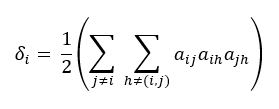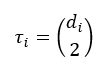# 1.1 - 8.10 - Unweighted, Undirected Network (BUN) - Teradata Vantage

## Teradata Vantage™ - Machine Learning Engine Analytic Function Reference

Product
Release Number
1.1
8.10
Release Date
October 2019
Content Type
Programming Reference
Publication ID
B700-4003-079K
Language
English (United States)

The local clustering coefficient was originally defined on an unweighted, undirected graph—also called a bi-directed network (BUN). A simple BUN has no self-loops and no multiple edges.

Let G = (V, E) be a simple BUN with a set of nodes (vertices) V and a set of edges E.

The degree d i of node i is the number of nodes in V that are adjacent to i. A complete subgraph of three nodes of G is called a triangle. This is the formula for the number of triangles of node i:where a ij = 1 if there is an edge from i to j; otherwise a ij = 0.

A triple Ƴ at a node i is a path of length two for which i is the center node. This is the formula for the maximum number of triples of node i:The maximum number of triples occurs when every neighbor of node i is connected to every other neighbor of node i.

This is the formula for the clustering coefficient for a node i with d i ≥ 2:

c i = δ i / τ i